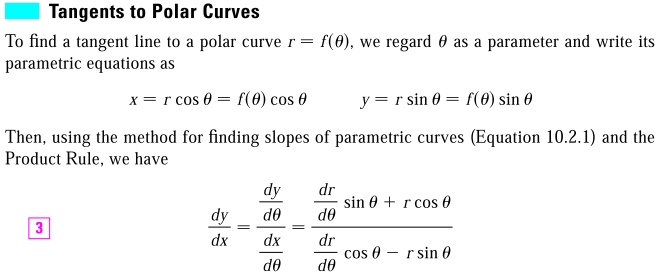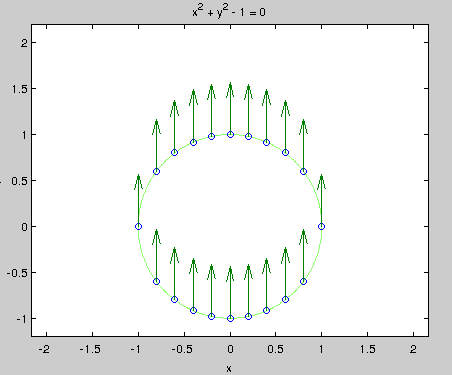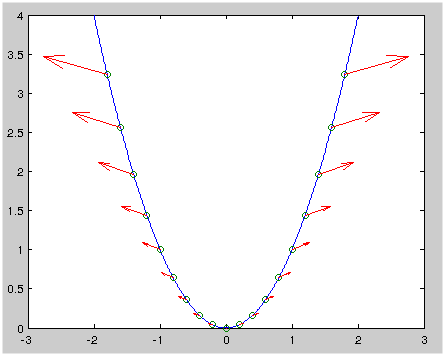## Slope in polar coordinates connected to rotation matrix

Slope in polar coordinates connected to rotation matrix

Here is how you can the tangent line slope, given a function in polar coordinates:Let's see it from a matrix algebra point of view:

\begin{align*} \begin{pmatrix} dx/d\theta \\ dy/d\theta \\ \end{pmatrix} &= \begin{pmatrix} \cos \theta & -\sin \theta \\ \sin \theta & \cos \theta \\ \end{pmatrix} \begin{pmatrix} dr/d\theta \\ r\\ \end{pmatrix} \\ &= R_{\theta} \begin{pmatrix} dr/d\theta \\ r\\ \end{pmatrix} \end{align*}

or, reversely:

\begin{align*} \begin{pmatrix} dr/d\theta \\ r\\ \end{pmatrix} &= R_{-\theta} \begin{pmatrix} dx/d\theta \\ dy/d\theta \\ \end{pmatrix} \end{align*}

The matrix on the left looks familiar, huh? It's just a rotation matrix!

$\begin{pmatrix} dr/d\theta \\ r\\ \end{pmatrix}$ deserves a closer look on its own right. $r$ is the distance from the origin, $dr/d\theta$ is the rate of deviation from the origin as $\theta$ varies. Take the circle $r = 1$ as an example, $r$ is constant here, so $dr/d\theta$ is always zero, and $\begin{pmatrix} dr/d\theta \\ r\\ \end{pmatrix}$ is a constant vector:Vector field describing ($dr/d\theta$, r) for the circle $r = 1$
Click to show code
ezplot('x^2 + y^2 - 1', [-1.2 1.2 -1.2 2.2])
axis equal
hold on
x1 = -1:.2:1
x2 = x1(2:(end-1))
x = horzcat(x1, x2)
y = horzcat(sqrt(1- x1.^2), -sqrt(1 - x2.^2))
scatter(x, y)
u = repmat(0, size(x))
v = repmat(1, size(x))
quiver(x, y, u, v)


Take the parabola $y = x^2$ as another example. Its polar form is $r = \tan\theta \sec\theta$.

\begin{align*} dr/d\theta &= (1 + \sin^2 \theta) / \cos^3 \theta \\ &= (r^3 + ry^2) / x^3 \\ \begin{pmatrix} dr/d\theta \\ r \\ \end{pmatrix} &= \begin{pmatrix} (r^3 + ry^2) / x^3 \\ r \\ \end{pmatrix} \end{align*}

where $r = \sqrt{x^2 + y^2}$. Both $dr/d\theta$ and $r$ goes to infinity when $\theta$ goes to $\pi / 2$, the ratio $\frac{dr/d\theta}{r}$ also goes to infinity, which means the vector \begin{pmatrix} dr/d\theta \\ r \\ \end{pmatrix} becomes more and more flat.

which in a vector field likes like this:Vector field describing ($dr/d\theta$, r) for the parabola $y = x^2$ (matlab code)
Click to toggle code (may have to try twice)
x = -2:.01:2
y = x.^2
plot(x, y)
hold on
xvec = -1.8:0.2:1.8
yvec = xvec.^2
scatter(xvec, yvec)
vvec = sqrt(xvec.^2 + yvec.^2)
uvec = (vvec.^3 + vvec .* yvec.^2) ./ xvec.^3
quiver(xvec, yvec, uvec, vvec)


A quick look confirms that when you rotate \begin{pmatrix} dr/d\theta \\ r \\ \end{pmatrix} by $\theta$, you get the tagent line at $\theta$, and as $\theta$ increases, the line gets more and more vertical, which is indeed true: $y' = 2x$.